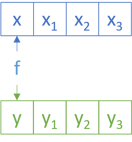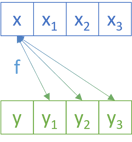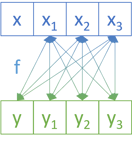The universal API for generic non-empty collections, with examples in C# and Haskell.

I was recently doing some work that required a data structure like a collection, but with the additional constraint that it should be guaranteed to have at least one element. I've known about Haskell's NonEmpty type, and how to port it to C# for years. This time I needed to implement it in a third language, and since I had a little extra time available, I thought it'd be interesting to pursue a conjecture of mine: It seems as though you can implement most (all?) of a generic data structure's API based on its catamorphism.

While I could make a guess as to how a catamorphism might look for a non-empty collection, I wasn't sure. A quick web search revealed nothing conclusive, so I decided to deduce it from first principles. As this article series demonstrates, you can derive the catamorphism from a type's isomorphic F-algebra.

The beginning of this article presents the catamorphism in C#, with an example. The rest of the article describes how to deduce the catamorphism. This part of the article presents my work in Haskell. Readers not comfortable with Haskell can just read the first part, and consider the rest of the article as an optional appendix.

### C# catamorphism #

This article will use a custom C# class called `NonEmptyCollection<T>`, which is near-identical to the `NotEmptyCollection<T>` originally introduced in the article Semigroups accumulate.

I don't know why I originally chose to name the class `NotEmptyCollection` instead of `NonEmptyCollection`, but it's annoyed me ever since. I've finally decided to rectify that mistake, so from now on, the name is `NonEmptyCollection`.

The catamorphism for `NonEmptyCollection` is this instance method:

```public TResult Aggregate<TResult>(Func<T, IReadOnlyCollection<T>, TResult> algebra)
{
}```

Because the `NonEmptyCollection` class is really just a glorified tuple, the `algebra` is any function which produces a single value from the two constituent values.

It's easy to fall into the trap of thinking of the catamorphism as 'reducing' the data structure to a more compact form. While this is a common kind of operation, loss of data is not inevitable. You can, for example, return a new collection, essentially doing nothing:

```var nec = new NonEmptyCollection<int>(42, 1337, 2112, 666);
var same = nec.Aggregate((x, xs) => new NonEmptyCollection<int>(x, xs.ToArray()));```

This `Aggregate` method enables you to safely find a maximum value:

```var nec = new NonEmptyCollection<int>(42, 1337, 2112, 666);
var max = nec.Aggregate((x, xs) => xs.Aggregate(x, Math.Max));```

```var nec = new NonEmptyCollection<int>(42, 1337, 2112, 666);
var average = nec.Aggregate((x, xs) => xs.Aggregate(x, (a, b) => a + b) / (xs.Count + 1.0));```

Both of these two last examples use the built-in Aggregate function to accumulate the `xs`. It uses the overload that takes a seed, for which it supplies `x`. This means that there's guaranteed to be at least that one value.

The catamorphism given here is not unique. You can create a trivial variation by swapping the two function arguments, so that `x` comes after `xs`.

### NonEmpty F-algebra #

As in the previous article, I'll use `Fix` and `cata` as explained in Bartosz Milewski's excellent article on F-algebras.

```data NonEmptyF a c = NonEmptyF { head :: a, tail :: ListFix a }

instance Functor (NonEmptyF a) where
fmap _ (NonEmptyF x xs) = NonEmptyF x xs```

Instead of using Haskell's standard list (`[]`) for the tail, I've used `ListFix` from the article on list catamorphism. This should, hopefully, demonstrate how you can build on already established definitions derived from first principles.

Since a non-empty collection is really just a glorified tuple of head and tail, there's no recursion, and thus, the carrier type `c` is not used. You could argue that going through all of these motions is overkill, but it still provides some insights. This is similar to the Boolean catamorphism and Maybe catamorphism.

The `fmap` function ignores the mapping argument (often called `f`), since the `Functor` instance maps `NonEmptyF a c` to `NonEmptyF a c1`, but the `c` or `c1` type is not used.

As was the case when deducing the recent catamorphisms, Haskell isn't too happy about defining instances for a type like `Fix (NonEmptyF a)`. To address that problem, you can introduce a `newtype` wrapper:

```newtype NonEmptyFix a =
NonEmptyFix { unNonEmptyFix :: Fix (NonEmptyF a) } deriving (Eq, Show, Read)```

You can define `Functor`, `Applicative`, `Monad`, etc. instances for this type without resorting to any funky GHC extensions. Keep in mind that, ultimately, the purpose of all this code is just to figure out what the catamorphism looks like. This code isn't intended for actual use.

A helper function makes it easier to define `NonEmptyFix` values:

```createNonEmptyF :: a -> ListFix a -> NonEmptyFix a
createNonEmptyF x xs = NonEmptyFix \$ Fix \$ NonEmptyF x xs```

Here's how to use it:

```ghci> createNonEmptyF 42 \$ consF 1337 \$ consF 2112 nilF
NonEmptyFix {
unNonEmptyFix = Fix (NonEmptyF 42 (ListFix (Fix (ConsF 1337 (Fix (ConsF 2112 (Fix NilF)))))))}```

While this is quite verbose, keep in mind that the code shown here isn't meant to be used in practice. The goal is only to deduce catamorphisms from more basic universal abstractions, and you now have all you need to do that.

At this point, you have two out of three elements of an F-Algebra. You have an endofunctor (`NonEmptyF a`), and an object `c`, but you still need to find a morphism `NonEmptyF a c -> c`. Notice that the algebra you have to find is the function that reduces the functor to its carrier type `c`, not the 'data type' `a`. This takes some time to get used to, but that's how catamorphisms work. This doesn't mean, however, that you get to ignore `a`, as you'll see.

As in the previous articles, start by writing a function that will become the catamorphism, based on `cata`:

```nonEmptyF = cata alg . unNonEmptyFix
where alg (NonEmptyF x xs) = undefined```

While this compiles, with its `undefined` implementation of `alg`, it obviously doesn't do anything useful. I find, however, that it helps me think. How can you return a value of the type `c` from `alg`? You could pass a function argument to the `nonEmptyF` function and use it with `x` and `xs`:

```nonEmptyF :: (a -> ListFix a -> c) -> NonEmptyFix a -> c
nonEmptyF f = cata alg . unNonEmptyFix
where alg (NonEmptyF x xs) = f x xs```

This works. Since `cata` has the type `Functor f => (f a -> a) -> Fix f -> a`, that means that `alg` has the type `f a -> a`. In the case of `NonEmptyF`, the compiler infers that the `alg` function has the type `NonEmptyF a c -> c1`, which fits the bill, since `c` may be the same type as `c1`.

This, then, is the catamorphism for a non-empty collection. This one is just a single function. It's still not the only possible catamorphism, since you could trivially flip the arguments to `f`.

I've chosen this representation because the arguments `x` and `xs` are defined in the same order as the order of `head` before `tail`. Notice how this is the same order as the above C# `Aggregate` method.

### Basis #

You can implement most other useful functionality with `nonEmptyF`. Here's the `Semigroup` instance and a useful helper function:

```toListFix :: NonEmptyFix a -> ListFix a
toListFix = nonEmptyF consF

instance Semigroup (NonEmptyFix a) where
xs <> ys =
nonEmptyF (\x xs' -> createNonEmptyF x \$ xs' <> toListFix ys) xs```

The implementation uses `nonEmptyF` to operate on `xs`. Inside the lambda expression, it converts `ys` to a list, and uses the `ListFix` `Semigroup` instance to concatenate `xs` with it.

Here's the `Functor` instance:

```instance Functor NonEmptyFix where
fmap f = nonEmptyF (\x xs -> createNonEmptyF (f x) \$ fmap f xs)```

Like the `Semigroup` instance, this `fmap` implementation uses `fmap` on `xs`, which is the `ListFix` `Functor` instance.

The `Applicative` instance is much harder to write from scratch (or, at least, I couldn't come up with a simpler way):

```instance Applicative NonEmptyFix where
pure x = createNonEmptyF x nilF
liftA2 f xs ys =
nonEmptyF
(\x xs' ->
nonEmptyF
(\y ys' ->
createNonEmptyF
(f x y)
(liftA2 f (consF x nilF) ys' <> liftA2 f xs' (consF y ys')))
ys)
xs```

While that looks complicated, it's not that bad. It uses `nonEmptyF` to 'loop' over the `xs`, and then a nested call to `nonEmptyF` to 'loop' over the `ys`. The inner lambda expression uses `f x y` to calculate the head, but it also needs to calculate all other combinations of values in `xs` and `ys`.First, it keeps `x` fixed and 'loops' through all the remaining `ys'`; that's the `liftA2 f (consF x nilF) ys'` part:Then it 'loops' over all the remaining `xs'` and all the `ys`; that is, `liftA2 f xs' (consF y ys')`.The two `liftA2` functions apply to the `ListFix` `Applicative` instance.

You'll be happy to see, I think, that the `Monad` instance is simpler:

```instance Monad NonEmptyFix where
xs >>= f =
nonEmptyF (\x xs' ->
nonEmptyF
(\y ys -> createNonEmptyF y \$ ys <> (xs' >>= toListFix . f)) (f x)) xs```

And fortunately, `Foldable` and `Traversable` are even simpler:

```instance Foldable NonEmptyFix where
foldr f seed = nonEmptyF (\x xs -> f x \$ foldr f seed xs)

instance Traversable NonEmptyFix where
traverse f = nonEmptyF (\x xs -> liftA2 createNonEmptyF (f x) (traverse f xs))```

Finally, you can implement conversions to and from the `NonEmpty` type from `Data.List.NonEmpty`:

```toNonEmpty :: NonEmptyFix a -> NonEmpty a
toNonEmpty = nonEmptyF (\x xs -> x :| toList xs)

fromNonEmpty :: NonEmpty a -> NonEmptyFix a
fromNonEmpty (x :| xs) = createNonEmptyF x \$ fromList xs```

This demonstrates that `NonEmptyFix` is isomorphic to `NonEmpty`.

### Conclusion #

The catamorphism for a non-empty collection is a single function that produces a single value from the head and the tail of the collection. While it's possible to implement a 'standard fold' (`foldr` in Haskell), the non-empty catamorphism doesn't require a seed to get started. The data structure guarantees that there's always at least one value available, and this value can then be use to 'kick off' a fold.

In C# one can define the catamorphism as the above `Aggregate` method. You could then define all other instance functions based on `Aggregate`.

Next: Either catamorphism.

### Wish to comment?

You can add a comment to this post by sending me a pull request. Alternatively, you can discuss this post on Twitter or somewhere else with a permalink. Ping me with the link, and I may respond.

#### Published

Monday, 07 August 2023 11:40:00 UTC

#### Tags

"Our team wholeheartedly endorses Mark. His expert service provides tremendous value."
Hire me!
Published: Monday, 07 August 2023 11:40:00 UTC